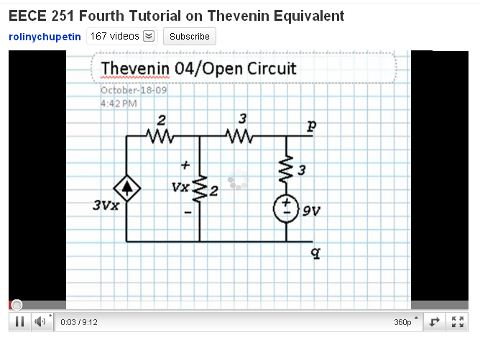# Fourth Tutorial on Thevenin EquivalentThis is Thevenin equivalent tutorial video part 4. This video shows how to calculate Thevenin equivalent with control source and an independent source using current loop. In this video, to calculate the Thevenin voltage Ref and V1 are used. First, the circuit is tested using open circuit test to obtain Voc. Then, to obtain Isc, the circuit is tested in short circuit test. After that the R Tcan be calculated by dividing Voc with Isc.